Start typing, then use the up and down arrows to select an option from the list.
2:30 minutes
Problem 95
Textbook Question

# Hess’s law can be used to calculate reaction enthalpies for hypothetical processes that can’t be carried out in the labo- ratory. Set up a Hess’s law cycle that will let you calculate ∆H° for the conversion of methane to ethylene: 2 CH4(g) → C2H4(g) + 2 H2(g) You can use the following information: 2 C2H6(g) + 7 O2(g) → 4 CO2(g) + 6 H2O(l) ∆H° = -3120.8 kJ CH4(g) + 2 O2(g) → CO2(g) + 2 H2O(l) ∆H° = -890.3 kJ C2H4(g) + H2(g) → C2H6(g) ∆H° = -136.3 kJ H2O(l) ∆H°f = -285.8 kJ/molVerified Solution
This video solution was recommended by our tutors as helpful for the problem above.
126views

### Watch next

Master Hess's Law with a bite sized video explanation from Jules Bruno

Start learning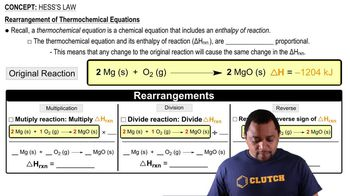03:11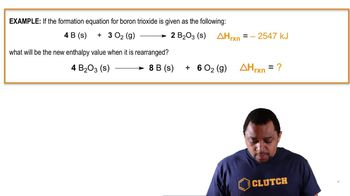01:39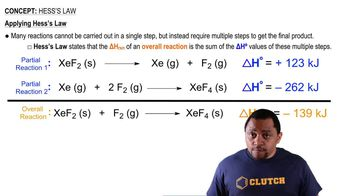02:03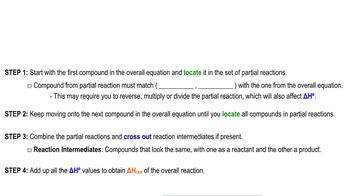05:43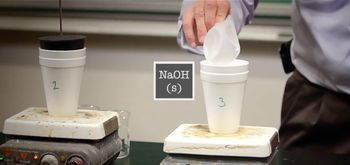07:46